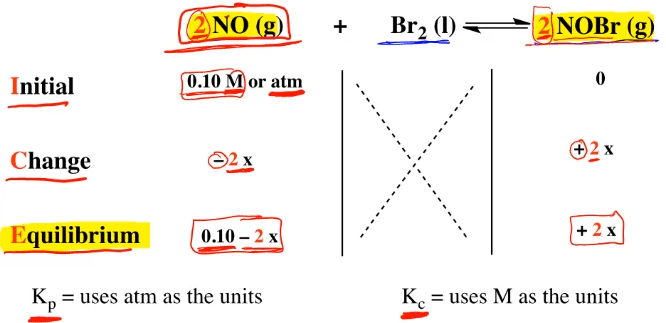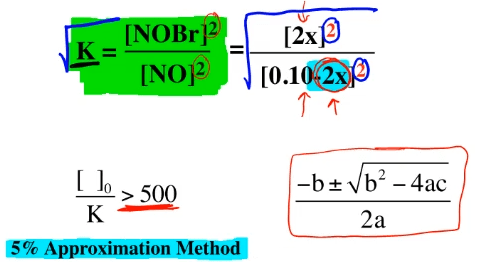Clutch Prep is now a part of Pearson
Ch.14 - Chemical EquilibriumWorksheetSee all chapters

# Equilibrium Expressions

See all sections
Sections
Chemical Equilibrium
ICE Chart
Le Chatelier's Principle
The Reaction Quotient
Equilibrium ExpressionsJules Bruno

# Equilibrium Expressions

When dealing with chemical equilibrium, there will come a time when you have to set up an ICE chart. An ICE chart should be used when we are missing more than one equilibrium amount for the compounds in our balanced equation.

## Writing equilibrium expressions

In this example question, we have 2 moles of nitrogen monoxide gas reacting with 1 mole of bromine liquid to produce 2 moles of NOBr gas. ICE stands for Initial Change Equilibrium. We’re given an initial amount for this reactant here. The units in an ICE chart can be in molarity if we’re dealing with case C because here C stands for concentration which is molarity or the units can be in atmospheres when we’re dealing with Kp. Here, p stands for pressure which in terms of units would be atmospheres.

We ignore Br2 because it’s a liquid. In an ICE chart, we ignore solids and liquids. We’re not given an initial amount for our product which is pretty normal. Here in this question, we have to look at these two compounds. Again, we’re ignoring bromine because it’s a liquid. Here I’m giving us the initial amount of the reactant. At equilibrium, I don’t give you any equilibrium amounts for either compound. We’re missing more than one equilibrium amount, Therefore, that’s why we’re setting up an ICE chart.

Remember, an ICE chart stands for Initial Change Equilibrium. Remember that reactants decrease over time to create products. We lose reactants to create products. Because we’re losing reactants, the sign will be negative. The coefficient here is 2 so that’s why it’s 2x. As we’re creating products, the change is positive because they’re increasing over time. The coefficient here is 2 so that’s why it’s 2x. You bring down everything so that’d be 0.10 - 2x and it’d be here 0 + 2x but we just ignore the zero. That would be our filled out ICE chart.From there, you would set up your equilibrium expression. Remember, K which is your equilibrium constant equals products over reactants. This represents our equilibrium expression. Again, we ignore solids and liquids in our ICE chart so the Br2 would be found nowhere within our equilibrium expression. Products over reactants, it’d be NOBr. Here, the coefficient is 2 so that becomes the power here divided by NO. The coefficient is 2 so that’s why it’s squared here as well. At equilibrium, we have these representing those compounds. That’s what we plug in to our equilibrium expression.

We’d solve for x here to help us figure out the equilibrium amounts for both of those compounds. We need to realize here that on the bottom, we can choose to ignore this minus 2x. We can check to see if it’s significant or not. To test to see if it’s significant or not, we use the 5% approximation method. You would just take your initial concentration which is this 0.10 and you would divide it by the equilibrium constant given to you in this question. Here, I don’t give us an equilibrium amount. Just realize that you would take the initial concentration of 0.10 divided by whatever the Kc or Kp is. If that ratio is greater than 500, then we would be able to ignore this minus 2x on the bottom. It would just drop out and we’d solve for x.

However, if I do the ratio and initial concentration divided by my equilibrium constant is not greater than 500, then I would keep this minus 2x and I’d have to use the quadratic formula which is right here to solve for x. Just remember, the 5% approximation is a way that we can cheat and avoid the quadratic formula. Another thing we can do is if both my top and bottom are both squared, I can simply take the square root to avoid the quadratic formula as well. Again, you have the 5% approximation method and also you have the square root method. If both the top and bottom are squared, you can just take the square root of both sides and solve for x that way and still avoid the quadratic formula. If neither one of these ways works, then we have to set up the quadratic formula and solve for x that way.Jules Bruno

Jules felt a void in his life after his English degree from Duke, so he started tutoring in 2007 and got a B.S. in Chemistry from FIU. He’s exceptionally skilled at making concepts dead simple and helping students in covalent bonds of knowledge.